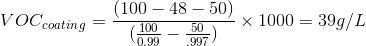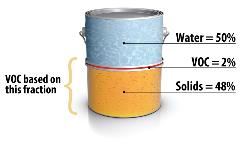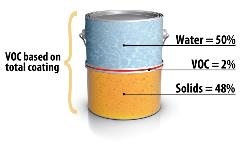Share:

## VOC Calculations

Regulatory and Actual VOC

## Calculating VOC Content of a Material

There is sometimes confusion over the terms VOC of coating/colorant/adhesive and VOC of material. The VOC of coating/colorant/adhesive is the same as the term “regulatory VOC,” which is equivalent to the term "VOC, less water and exempts." The VOC of material is the same as the term "actual VOC," which is equivalent to the term "VOC, including water and exempts."

The regulatory VOC calculates the VOC less exempts and water, which is a more complicated calculation than the actual VOC, in that it subtracts the volume of water and the volume of exempt compounds from the volume of material, in the denominator. The calculation was derived to express the VOC emitted per volume of coating solids to eliminate the effect of dilution. Dilution with water or exempt solvents would reduce the VOC-to-paint-volume ratio while maintaining a constant VOC-to-paint/adhesive-solids ratio. This is important because those materials are applied at a certain film thickness so dilution would result in a larger volume of the material being applied to achieve the same film thickness. For conventional solvent based products with no exempt compounds, the two values are always the same.

For waterborne coatings and coatings containing exempt compounds, the VOC of coating is always the higher of the two values. The VOC limits listed in the Table of Standards (PDF, 35kb) refer to the VOC of coating. The only exception is for low solids coatings where the purpose of the coating is not to build film thickness. Low solids coatings, which are defined as coatings containing less than one pound of solids per gallon of coating, are regulated by the VOC of material. VOC emissions, including the emissions fee in Rule 314, are also calculated based on the VOC of material.

The VOC Calculator (XLS, 90kb) is a spreadsheet that will calculate both VOC values based on the solids, water content and density of the material.

## Sample Coating Calculation:

Density= 0.99 g/mL
Water= 50% by weight
Density of water= 0.997 g/mL
Solids= 48% by weight

## VOC of Coating Calculation (Regulatory VOC, less water and exempt compounds): Remove the volume solid from denominator  Volume Solids= [Weight Solids]/[Density]## VOC of Material Calculation (Actual VOC):## You may also like ...Related Topics

bparrack@aqmd.gov
(909) 396-3071